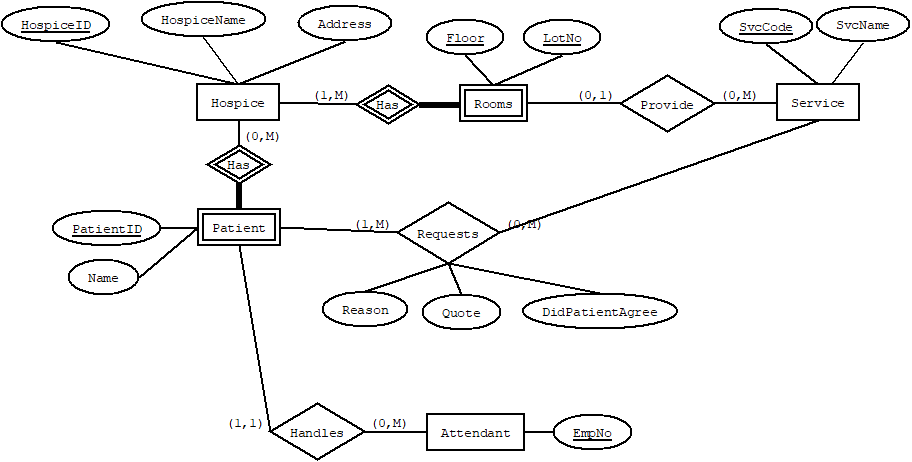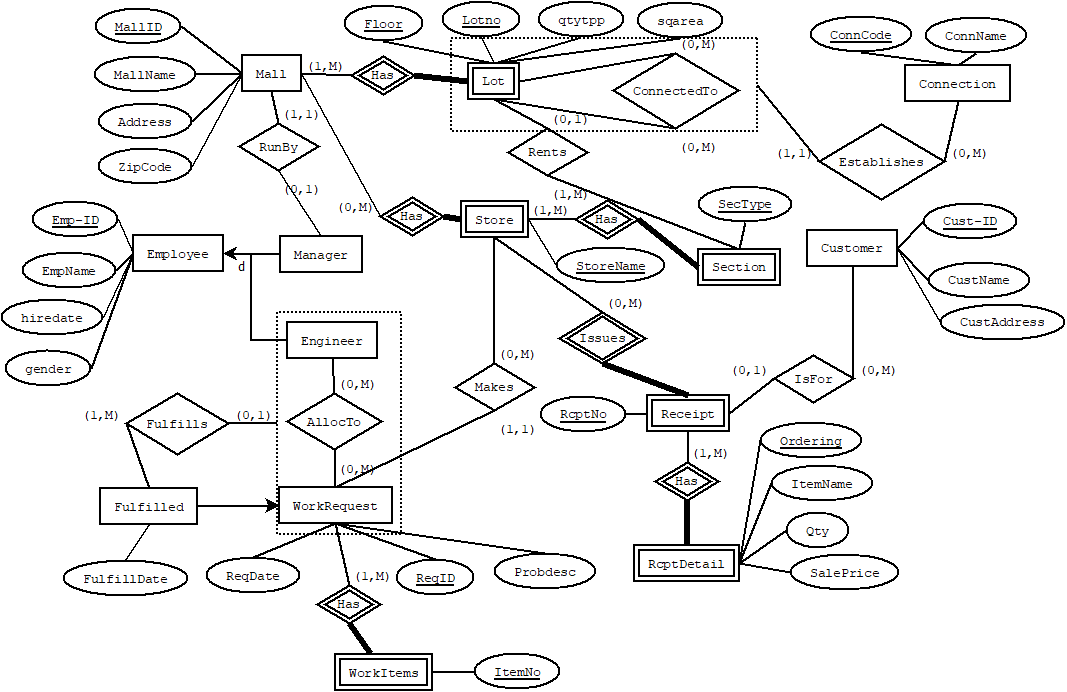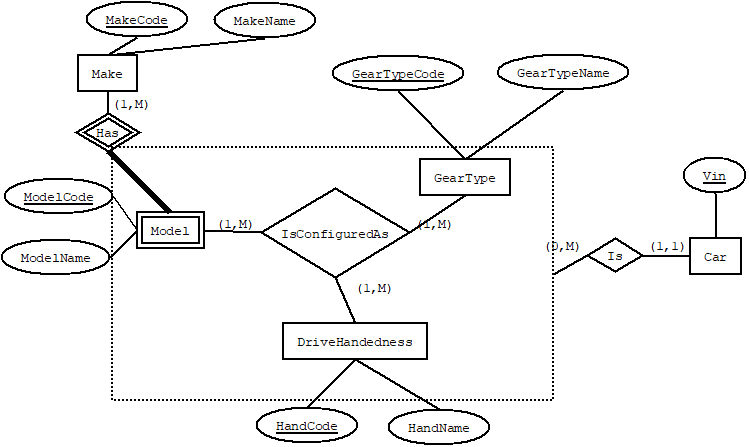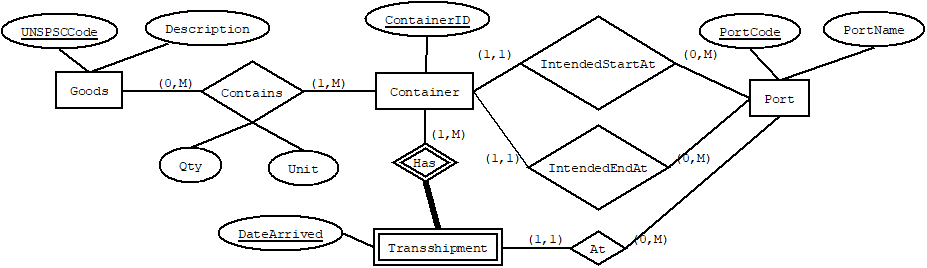# Introduction

Decomposition is a process of applying transformation rules to ER diagrams to make them into relational database tables. This is a topic of particular interest to me, because most instructors and textbooks use the transformation rules specified in the pioneering work on the subject- Teorey et al. 1986. The problem is the field has moved on since then. This section provides a more modern approach to decomposition.

The decomposition rules I employ are found here. These serve as a useful handout to students, explaining each rule in detail.

The current contents are:

Any questions or comments should be directed to: The creator's email

# Binary Decomposition

This video introduces the basic rules for decomposition where we have [entity set]-<relationship set>-[entity set]. Note especially for the 1:1 and 1:M relationship types, these are not the rules you'll find in most textbooks. I go through each rule in detail and explain why we employ each rule.

The exercise described in the video is below.Any questions or comments should be directed to: The creator's email

# Unary Decomposition

This pair of videos introduces the rules for decomposition where we have unary relationship sets. The first video explains the rules.

This second video walks through an exercise.

The exercise described in the video is below.Any questions or comments should be directed to: The creator's email

# N-ary Decomposition

This video discusses decomposition when a relationship set is connected to more than two entity sets.

The exercise described in the video is below.Any questions or comments should be directed to: The creator's email

# Decomposition vs. Normalization

Following decomposition rules is much easier than normalization. So why do normalization at all? This video describes situations where decomposition gives an incompletely normalized database.

The exercise described in the video is below.Any questions or comments should be directed to: The creator's email